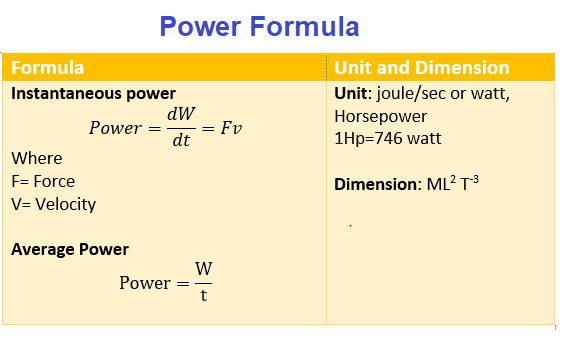# Power Definition, Formula ,Calculation ,Examples

## (12) Power

• Power is defined as rate of doing the work
• if ΔW amount of work is done in time interval Δt ,the instantaneous power delivered will be
$P=\frac {\Delta W}{ \Delta t}$
or
$P=\frac {dW}{dt}$
• For total work done W in total time t,then average power is defined
$P_{avg}=\frac {W}{t}$
• If Power (P) does not vary with time ,then $P=P_{avg}$
• the instantaneous power can also be written as
$P=\frac {dW}{dt}$
Now as dW=Fdx
$P=\frac {F dx}{dt}$
$P = F \frac {dx}{dt}$
Now velocity is given as $v= \frac {dx}{dt}$
Therefore,
$P =F.v$
This can be written in vector form as
P=F.v
This is scalar dot product of Force and velocity vector.
If the Force and velocity vector are at angle,then
$P= Fvcos \theta$
• SI unit of power is joule/sec also called watt
• Power, like work and energy, is a scalar quantity. Its dimensions are $[ML^2T^{-3}]$.
• Another unit of power is horsepower(hp)
1Hp=746 wattExample-1
An elevator can carry a maximum load of 1000 kg (elevator + passengers) is moving up with a constant speed of 2 m/s. The frictional forces opposing the motion is 4000 N. Determine the minimum power delivered by the motor to the elevator in watts ?
Solution
The downward force on the elevator is
$F = m g + f_{fric}$
$f = (1000 \times 10) + 4000 = 14000 N$
The motor must supply enough power to balance this force. Hence,
$P = F. v = 14000 \times 2 = 28000 W$

Example-2
A truck of mass 1000 kg accelerates on the level road from 10 m/s to 20 m/s in 5 sec. The frictional force present is 4000N. Find the average power delivered by the engine.?
Solution
acceleration of truck = $\frac {20 -10}{5} = 2 m/s^2$
So total force delivered by the engine = $ma + f_{fric} = 1000 \times 2 + 4000 = 6000 N$
Average power = F X average velocity = 6000 X 30 = 18000W